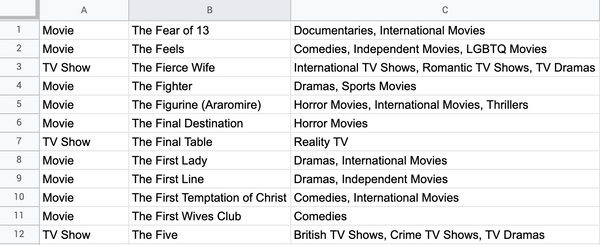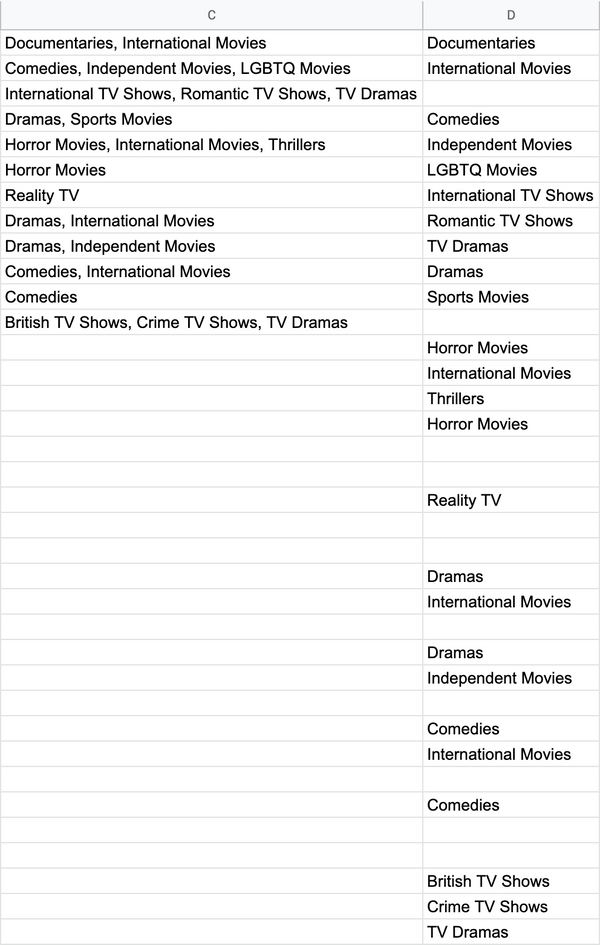# Getting unique categories in column with comma separated categories

I often get data sets or survey responses where there is a column for categories, and in each cell the categories are separated by a comma. You might find something like this for movies data:

``````comedy, action, kids, animated
romance, action
documentaries, sports
``````

If you had many rows with many categories then it wouldn't be so clear what all the categories are; and it's always more accurate to use a systematic approach than to eye-ball it.

## Here's my approach

With the sample data, I want to get all the categories in column Cnetflix

### Final formula

For those who don't want the walk-thru here's the final formula I used in cell D1

``````=SORT(UNIQUE(FLATTEN(ARRAYFORMULA(SPLIT(C1:C12, ", ", FALSE)))))
``````

## The formula walk-thru

### 1. Separate out the categories by comma

In cell D1 input the formula

``````=ARRAYFORMULA(SPLIT(C1:C12, ", ", FALSE))
``````

Using the `SPLIT` function we can split out the categories by comma. I'm using the optional `FALSE` flag for `split_by_each` so that I'm splitting by the comma and the space, otherwise the second category and beyond will start with a space. I could have instead leverage the TRIM function instead. I'm splitting all 12 rows with just one formula by using `ARRAYFORMULA`, I didn't want to fill down, you'll see why later. Notice that the categories fill up the columns D, E, Fnetflix

### 2. Flatten out columns D, E, F into one column

Update the cell in D1 to this one

``````=FLATTEN(ARRAYFORMULA(SPLIT(C1:C12, ", ", FALSE)))
``````

I can't always be certain how many columns the categories will get split out into. In the sample data the max number of categories per movie/show appears to be 3, but there could be more or less if I had a different data set. That's why I use the `FLATTEN` formula so all the categories are in one column. This is also why I used `ARRAYFORMULA`: the `FLATTEN` formula can take all the split out categories as the range (I didn't have to explicitly say the range is columns D, E, F). Now all the categories are in column D, but there are duplicatesnetflix

### 3. Get unique categories and sort them alphabetically

Update the cell in D1 to this one

``````=SORT(UNIQUE(FLATTEN(ARRAYFORMULA(SPLIT(C1:C12, ", ", FALSE)))))
``````

The `UNIQUE` formula takes the results from the `FLATTEN` formula (everything you see in column D in the image above) and only shows the unique categories. Then I sorted that list alphabetically by wrapping the `SORT` formula around everything. Now column D shows all the unique categories for the sample datanetflix